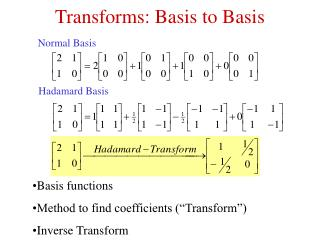DownloadDownload PresentationTransforms: Basis to Basis

# Transforms: Basis to Basis

Télécharger la présentation## Transforms: Basis to Basis

- - - - - - - - - - - - - - - - - - - - - - - - - - - E N D - - - - - - - - - - - - - - - - - - - - - - - - - - -
##### Presentation Transcript

1. Hadamard Basis Transforms: Basis to Basis Normal Basis • Basis functions • Method to find coefficients (“Transform”) • Inverse Transform

2. Basis for a Vector Space • Every vector in the space is a linear combination of basis vectors. • n independent vectors from an nth dimensional vector space form a basis. • Orthogonal Basis: Every two basis vectors are Orthogonal. • Orthonormal Basis: The absolute value of all basis vectors is 1.

3. Phase: Absolute Value: i Complex Numbers R b  a

4. Fourier: Fourier Spectrum Fourier Phase Fourier: Fourier Spectrum

5. Complexity: O(N2) (106 1012) FFT: O(N logN) (106 107) Discrete Fourier Transform Fourier Transform Inverse Fourier Transform

6. 2D Discrete Fourier Fourier Transform Inverse Fourier Transform

7. Display Fourier Spectrum as Picture 1. Compute 2. Scale to full range 3. Move (0,0) to center of image (Shift by N/2) Example for range 0..10: Original f 0 1 2 4 100 Scaled to 10 0 0 0 0 10 Log (1+f) 0 0.69 1.01 1.61 4.62 Scaled to 10 0 1 2 4 10

8. Decomposition

9. Decomposition (II) • 1-D Fourier is sufficient to do 2-D Fourier • Do 1-D Fourier on each column. On result: • Do 1-D Fourier on each row • (Multiply by N?) • 1-D Fourier Transform is enough to do Fourier for ANY dimension

10. Derivatives I Inverse Fourier Transform

11. Derivatives II • To compute the x derivative of f (up to a constant): • Computer the Fourier Transform F • Multiply each Fourier coefficient F(u,v) by u • Compute the Inverse Fourier Transform • To compute the y derivative of f (up to a constant): • Computer the Fourier Transform F • Multiply each Fourier coefficient F(u,v) by v • Compute the Inverse Fourier Transform

12. Translation

13. Periodicity & Symmetry

14. Rotation

15. Linearity

16. Convolution Theorem Convolution by Fourier: Complexity of Convolution: O(N logN)

17. High-Pass Filtering Band-Pass Filtering Filtering in the Frequency Domain Filtered Fourier Filtered Picture Fourier Filter Picture Low-Pass Filtering

18. Low Pass: Frequency & Image • (0 0 1 1 0 0)  Sinc • (0 0 1 1 0 0) * (0 0 1 1 0 0 ) = (0 1 2 1 0 0)  Sinc2 • (0 1 4 6 4 1 0) = (0 0 1 1 0 0 ) 4  Sinc4 • Fourier (Gaussian)  Gaussian

19. Image: · = = · T Fourier: = * 1/T = * 1/T Continuous Sampling